#### Chapter 20 Surface Area and Volume of a Right Circular Cone R.D. Sharma Solutions for Class 9th Exercise 20.1

Exercise 20.1

1. Find the curved surface area of a cone, if its slant height is 60 cm and the radius of its base is 21 cm.

Solution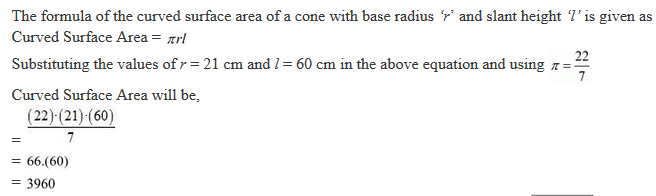2. The radius of a cone is 5 cm and vertical height is 12 cm. Find the area of the curved surface.

Solution3. The radius of a cone is 7 cm and area of curved surface is 176 cm2. Find the slant height.

Solution4. The height of a cone is 21 cm. Find the area of the base if the slant height is 28 cm.

Solution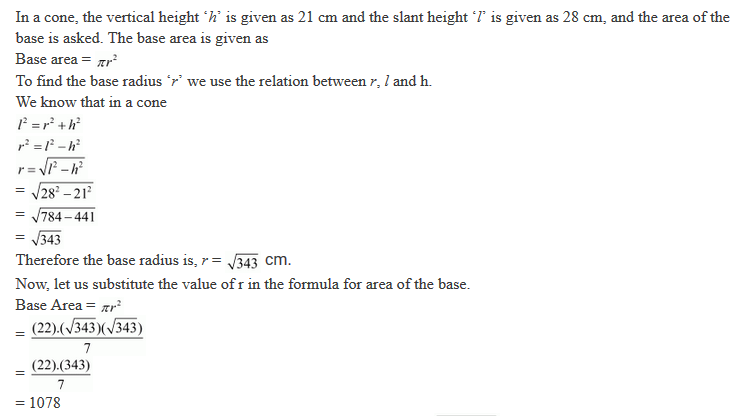5. Find the total surface area of a right circular cone with radius 6 cm and height 8 cm.

Solution6. Find the curved surface area of a cone with base radius 5.25 cm and slant height 10 cm.

Solution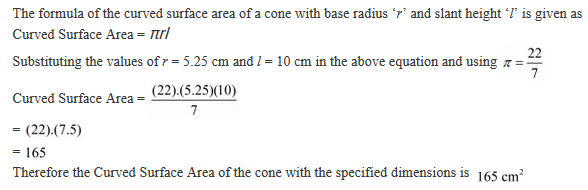7. Find the total surface area of a cone, if its slant height is 21 m and diameter of its base is 24 m.

Solution8. The area of the curved surface of a cone is 60$\mathrm{\pi }$cm2. If the slant height of the cone be 8 cm, find the radius of the base .

Solution9. The curved surface area of a cone is 4070 cm2 and its diameter is 70 cm. What is its slant height? (Use $\mathrm{\pi }$ = 22/7).

Solution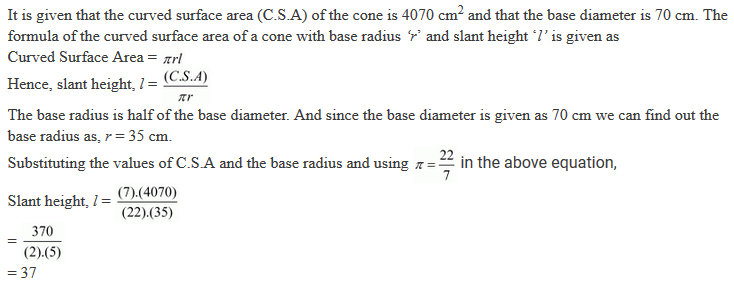10. The radius and slant height of a cone are in the ratio of 4 : 7. If its curved surface area is 792 cm2, find its radius. (Use $\mathrm{\pi }$ = 22/7).

Solution11. A joker's cap is in the form of a right circular cone of base radius 7 cm and height 24 cm. Find the area of the sheet required to make 10 such caps.

Solution12. Find the ratio of the curved surface areas of two cones if their diameters of the bases are equal and slant heights are in the ratio 4 : 3.

Solution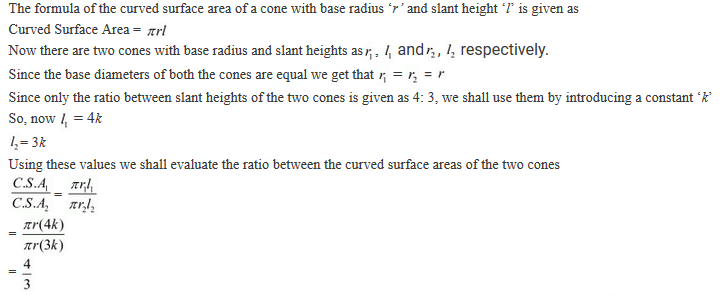13. There are two cones. The curved surface area of one is twice that of the other. The slant height of the later is twice that of the former. Find the ratio of their radii.

Solution14. The diameters of two cones are equal. If their slant heights are in the ratio 5 : 4, find the ratio of their curved surfaces.

Solution15. Curved surface area of a cone is 308 cm2 and its slant height is 14 cm. Find the radius of the base and total surface area of the cone.

Solution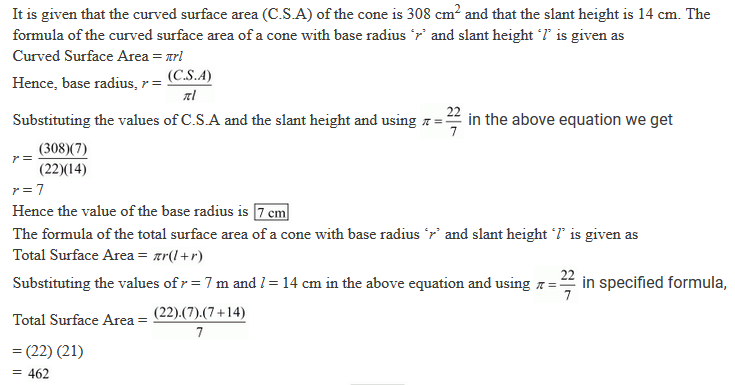16. The slant height and base diameter of a conical tomb are 25 m and 14 m respectively. Find the cost of white-washing its curved surface at the rate of Rs 210 per 100 m2.

Solution17. A conical tent is 10 m high and the radius of its base is 24 m. Find the slant height of the tent. If the cost of 1 m2 canvas is Rs 70, find the cost of the canvas required to make the tent.

Solution18. A tent is in the form of a right circular cylinder surmounted by a cone. The diameter of cylinder is 24 m. The height of the cylindrical portion is 11 m while the vertex of the cone is 16 m above the ground. Find the area of the canvas required for the tent.

Solution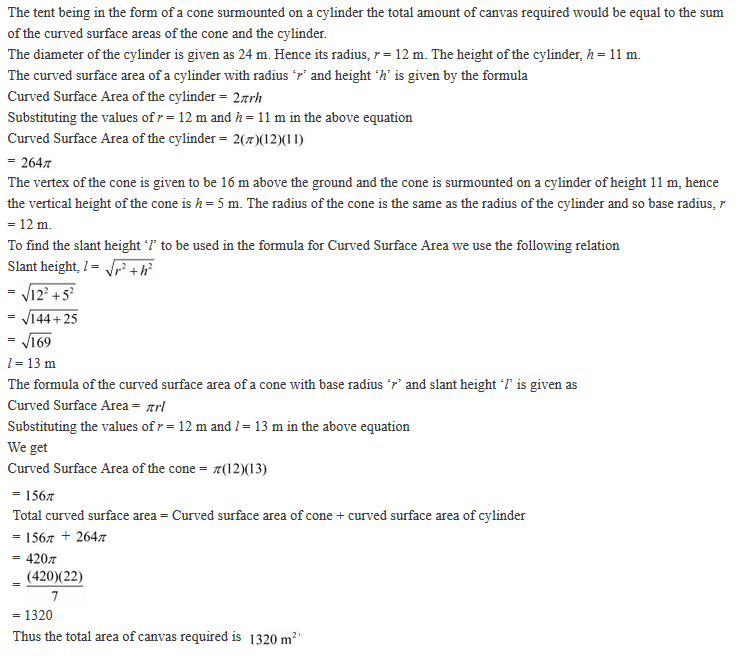19. A circus tent is cylindrical to a height of 3 metres and conical above it. If its diameter is 105 m and the slant height of the conical portion is 53 m, calculate the length of the canvas 5 m wide to make the required tent.

Solution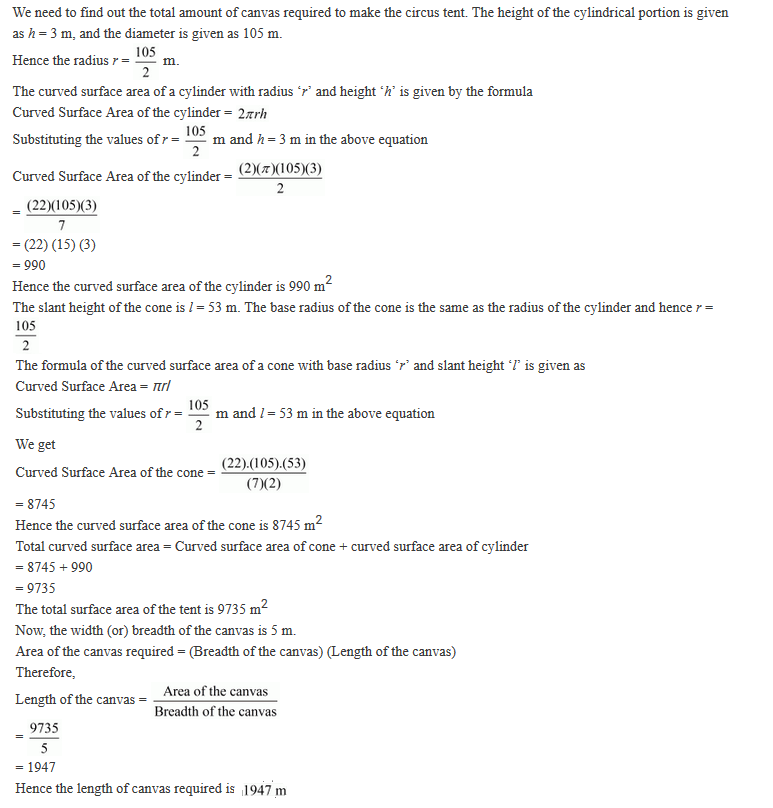20.  The circumference of the base of a 10 m height conical tent is 44 metres. Calculate the length of canvas used in making the tent if width of canvas is 2 m. (Use π = 22/7).

Solution21. What length of tarpaulin 3 m wide will be required to make a conical tent of height 8 m and base radius 6 m ? Assume that the extra length of material will be required for stitching margins and wastage in cutting is approximately 20 cm (Use π = 3.14)

Solution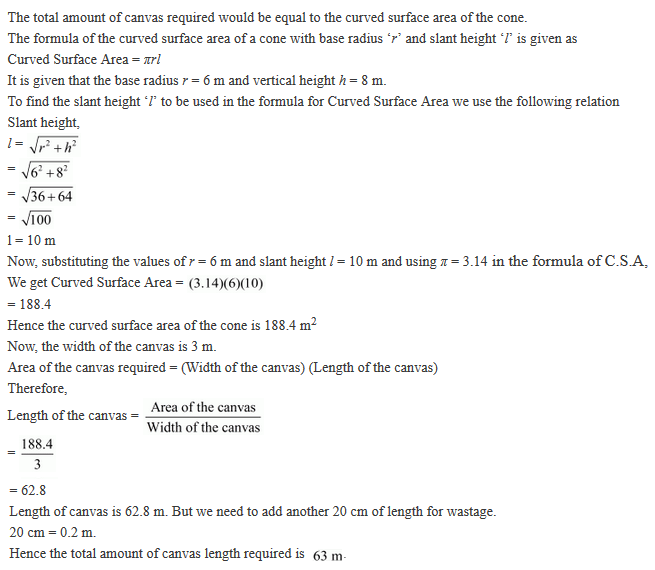22. A bus stop is barricated from the remaining part of the road, by using 50 hollow cones made of recycled card-board. Each cone has a base diameter of 40 cm and height 1 m. If the outer side of each of the cones is to be painted and the cost of painting is Rs 12 per m2. What will be the cost of painting all these cones. (use π = 3.14 and √1.04 = 1.02)

Solution23. A cylinder and a cone have equal radii of these bases and equal heights. If their curved surface areas are in the ratio 8:5, show that the radius of each is to the height of each as 3:4.

Solution